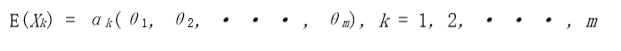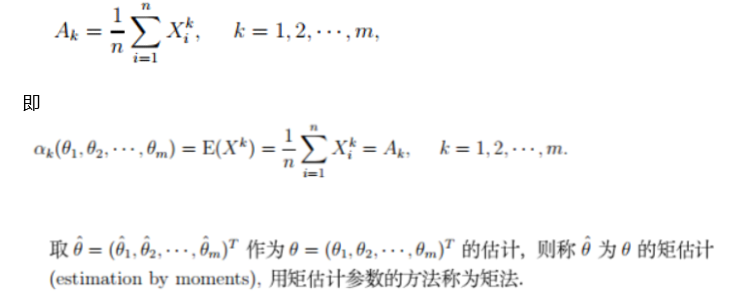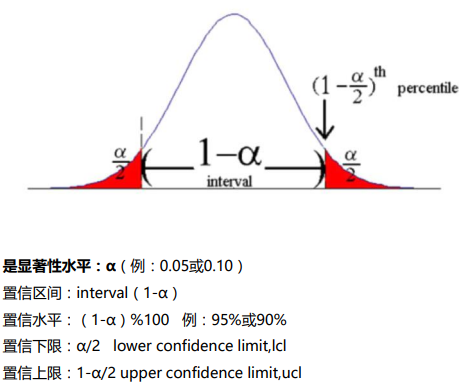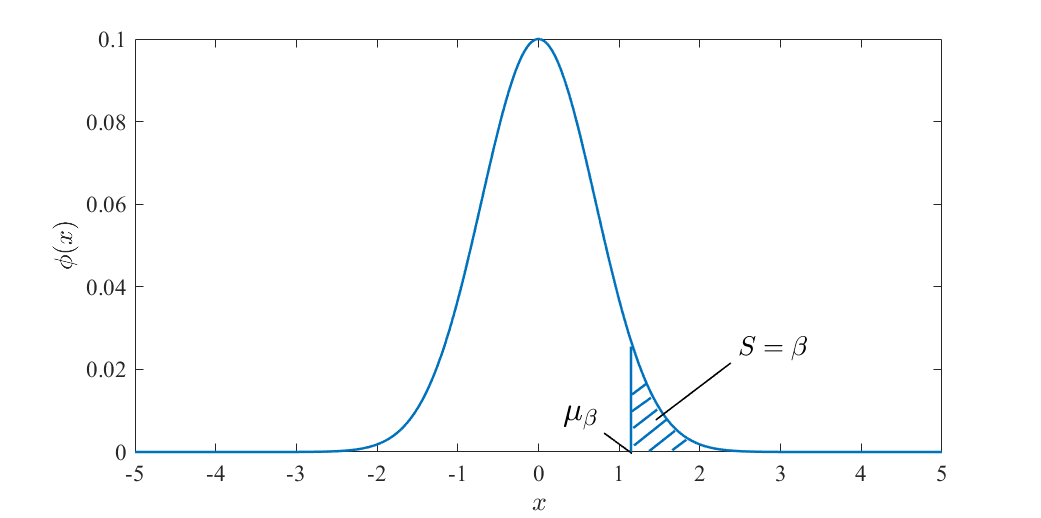• ## 什么是参数估计？

千次阅读 2020-10-20 20:06:51
参数估计(parameter estimation) 参数估计属于统计推断的范畴，是根据从总体中抽取的样本估计总体分布中包含的未知参数的方法。 统计推断是数理统计研究的核心问题，是指根据样本对总体分布或分布的数字特征等作出...
参数估计(parameter estimation)
目录
参数估计(parameter estimation)
点估计（point estimation）
矩估计法（method  of  moments）,
区间估计（interval estimation）
参数估计属于统计推断的范畴，是根据从总体中抽取的样本估计总体分布中包含的未知参数的方法。 统计推断是数理统计研究的核心问题，是指根据样本对总体分布或分布的数字特征等作出合理的推断。 参数估计分为：点估计、区间估计
点估计（point estimation）
点估计是依据样本估计总体分布中所含的未知参数或未知参数的函数。通常它们是总体的某个特征值，如数学期望、方差和相关系数等。点估计问题就是要构造一个只依赖于样本的量，作为未知参数或未知参数的函数的估计值。例如，设一批产品的废品率为θ。为估计θ，从这批产品中随机地抽出n 个作检查，以X记其中的废品个数，用X/n估计θ，这就是一个点估计。
构造点估计常用方法：
矩估计法：用样本矩估计总体矩，比如：用样本均值估计总体均值。最大似然估计法：于1912年由英国统计学家R.A.费希尔提出，利用样本分布密度构造似然函数来求出参数的最大似然估计。最小二乘法：主要用于线性统计模型中的参数估计问题。比如：Y=a0+a1X的参数估计就可以用最小乘法。贝叶斯估计法：基于贝叶斯学派的观点而提出的估计法。可以用来估计未知参数的估计量很多，于是产生了怎样选择一个优良估计量的问题。首先必须对优良性定出准则，这种准则是不唯一的，可以根据实际问题和理论研究的方便进行选择。优良性准则有两大类:一类是小样本准则，即在样本大小固定时的优良性准则;另一类是大样本准则，即在样本大小趋于无穷时的优良性准则。最重要的小样本优良性准则是无偏性及与此相关的一致最小方差无偏估计，其次有容许性准则， 最小化最大准则，最优同变准则等。大样本优良性准则有相合性、最优渐近正态估计和渐近有效估计等。
点估计能够明确告知人们“未知参数是多少”，但不能反映估计的可信程度。
矩估计法（method  of  moments）,
矩估计法也称"矩法估计"，原理是用样本矩作为相应的总体矩估计来求出估计量的方法，其思想是如果总体中有 K个未知参数，可以用前 K阶样本矩估计相应的前k阶总体矩，然后利用未知参数与总体矩的函数关系，求出参数的估计量。 矩法估计一般求的是一阶原点矩和二阶中心矩。
假设总体X的k阶原点矩：令总体的k阶原点矩等于它样本的k阶原点矩注：矩法相比于极大似然法、最小二乘法，效率很低。目前很少使用。

区间估计（interval estimation）
区间估计是依据抽取的样本，根据一定的正确度与精确度的要求，构造出适当的区间，作为总体分布的未知参数或参数的函数的真值所在范围的估计。
例如人们常说的有百分之多少的把握保证某值在某个范围内，即是区间估计的最简单的应用。
求置信区间常用的三种方法:
利用已知的抽样分布。利用区间估计与假设检验的联系。利用大样本理论。
区间估计可以告知置信区间范围，但不能直接告知人们“未知参数是多少”。
置信区间
区间估计(interval estimation)是从点估计值和抽样标准误出发，按给定的概率值建立包含待估计参数的区间。其中这个给定的概率值称为置信度或置信水平(confidence level)，这个建立起来的包含待估计参数的区间称为置信区间(confidence interval)，指总体参数值落在样本统计值某一区内的概率。
所谓置信水平就是给出一个区间的信心，这个信心以概率来表示，绝大多数情况下取 0.95，表示你对所估计的总体参数有95%的信心落在你所给的区间内。通常置信水平以1-α表 示，α称为显著性水平。
置信区间的建立就与中心极限定理和抽样分布有关了，在给定置信度的条件下，置信区间的宽度决定于抽样分布。 建立置信区间的意思是在设定的置信水平(如取0.95)下，总体参数落在这个区间的概率为 0.95，大致的理解是如果抽100次样，建立100个置信区间，大约95个区间包含总体参数，约5个区间不包含总体参数(注意不是一定有5个，可能会多，也可能会少)。
划定置信区间的两个数值分别称为置信下限(lower confidence limit,lcl)和置信上限(upper confidence limit,ucl)置信区间最主要的应用是用于假设检验。
展开全文算法
• ## 浅谈参数估计

千次阅读 2019-09-03 09:38:34
参数估计是推断统计的重要内容之一,它是在抽样及抽样分布的基础上,根据样本统计量来推断所关心的总体参数. 估计量 如果能够掌握总体的全部数据,那么只需要作一些简单的统计描述,就可以得到所关心的总体特征，比如，...
贾俊平<统计学>阅读笔记!

参数估计(parameter estimation)和假设检验（hypothesis testing）是统计推断的两个组成部分，它们都是利用样本对总体进行某种推断.但推断的角度不同。
参数估计讨论的是用样本统计量估计总体参数的方法.总体参数产在估计前是未知的。
而在假设检验中，则是先对产的值提出一个假设，然后利用样本信息去检验这个假设是否成立。
参数估计
参数估计是推断统计的重要内容之一,它是在抽样及抽样分布的基础上,根据样本统计量来推断所关心的总体参数.
如果能够掌握总体的全部数据,那么只需要作一些简单的统计描述,就可以得到所关心的总体特征，比如，总体均值、方差、比例，等。
但现实情况比较复杂，有些现象的范围比较广，不可能对总体中的每个单位都进行测定。或者，有些总体的个数很多，不可能也没必要一一测定。这就需要从总体中抽取一部分个体进行调查，进而利用样本提供的信息来推断总体的特征。
参数估计就是用样本统计量去估计总体的参数。比如：
用样本均值

x

x

直接作为总体均值

μ

\mu

的估计值，用样本比例

p

p

直接作为总比例

π

\pi

的估计值，用样本方差

s

2

s^2

直接作为总体方差

σ

2

\sigma^2

的估计值，等等。
如果，将总体参数笼统的用一个符号

θ

\theta

来表示，而用于估计总体参数的统计量用统计量

θ

\theta

估计量
在参数估计中，用来估计总体参数的统计量称为估计量，用符号

θ

−

\theta^-

表示。样本均值，样本比例、样本方差，等都可以是一个估计量。

样本估计量是样本的一个函数.（这句话一定要理解！！）
以样本平均数为例，它是总体平均数的一个估计量，如果按照相同的样本容量，相同的抽样方式，反复地抽取样本，每次可以计算一个平均数，所有可能样本的平均数所形成的分布，就是样本平均数的抽样分布。

估计值
而，根据一个具体的样本计算出来的估计量的数值，称为估计值。
参数估计的方法有两种：点估计和区间估计
1 点估计
点估计就是用样本统计量

θ

−

\theta^-

的某个取值，直接作为总体参数

θ

\theta

的估计值。
比如，假定要估计一个班学生考试成绩的平均分，根据抽出的一个随机样本计算的平均分数为80分，用80分作为全班平均考试分数的一个估计值，这就是点估计。
再比如，若要估计一批产品的合格率，根据抽样结果，合格率为96%，将96%直接作为这批产品合格率的估计值，这也是一个点估计。
点估计的问题1
虽然，在重复抽样条件下，点估计的均值可望等于总体均值。

比

如

，

E

(

x

−

)

=

μ

比如，E(x^-)=\mu

但，由于样本是随机的，抽出一个具体的样本得到的估计值很可能不同于总体均值。
所以，在用点估计值代表总体参数值的同时，还必须给出点估计值的可靠性，也就是说，必须能说出点估计值与总体参数的真实值的接近程度。
但，一个点估计值的可靠性是由它的抽样标准误差来衡量的，这表明一个具体的点估计值无法给出估计的可靠性的度量，因此，就不能完全依赖于一个点估计值，而是围绕点估计值构造总体参数的一个区间。这就是区间估计。
2 区间估计
在点估计的基础上，给出总体参数估计的一个区间范围，该区间由样本统计量加减估计误差而得到。

抽样误差：
由抽样的随机性引起的样本结果与总体真值之间的误差
标准误差：
衡量抽样误差大小的尺度，是样本统计量的标准差，反映用样本统计量去估计总体参数时，可能出现的平均“差错”
标准差VS标准误差：
标准差：反映样本中的元素对样本均值的离散程度，衡量个体间变异大小 标准误差：反映样本均值对总体均值的变异程度，从而衡量抽样误差的大小 随着样本量n的增大，标准差趋向某个稳定值，即样本标准差s越接近总体标准差σ，而标准误则随着样本量n的增大逐渐减小，即样本均值x越接近总体均值μ

置信区间
在区间估计中，由样本统计量所构造的总体参数的估计区间，称为置信区间。
其中，区间的最小值称为置信下限，最大值称为置信上限。
由于统计学家在某种程度上，确信这个区间会包含真正的总体参数，所以给它取名为置信区间。
置信水平
将构造置信区间的步骤重复很多次，置信区间包含总体参数真值的次数所占的比例称为置信水平。表示为

(

1

−

α

∗

100

)

(1-\alpha *100)

，

α

\alpha

是总体参数未在区间内的比例。
常用的置信水平值有 99%, 95%, 90%，相应的

α

\alpha

为0.01，0.05，0.10。评价估计量的标准
参数估计，是用样本估计量

θ

−

\theta^-

作为总体参数

θ

\theta

的估计。
实际上，用于估计

θ

\theta

的估计量有很多，比如，可以用样本均值作为总体均值的估计量，也可以用样本中位数作为总体均值的估计量，等等。
那么，究竟用样本的哪种估计量作为总体参数的估计呢？
自然要用估计效果最好的那种估计量。
什么样的估计量才算是一个好的估计量呢？
这就需要由一定的评价标准，统计学家给出了评价估计量的一些标准，主要有以下几个：
1 无偏性
估计量抽样分布的数学期望等于被估计的总体参数。无偏性说明，不同的样本得到的

θ

−

\theta^-

不同，可能大于

θ

\theta

，也可能小于

θ

\theta

，多次抽样时，

θ

−

\theta^-

的平均值与真实值

θ

\theta

一致。一个好的估计量就某一个具体的估计值而言，可能不等于总体参数值，但平均地看有向估计的参数集中的趋势。
2 有效性
一个无偏的估计量并不就意味着它非常接近被估计的参数，它还必须与总体参数的离散程度比较小。
有效性，是指对同一总体参数的两个无偏估计量，有更小标准差的估计量更有效。3 一致性
随着样本量的增大，估计量的值越来越接近被估计的总体参数。换言之，一个大样本给出的估计量要比一个小样本给出的估计量更接近总体的参数。展开全文• ## 参数估计

千次阅读 2018-10-10 23:31:28
一、参数估计内容 1.参数估计的requisites   我默认大家已经掌握了基本的概率论知识，例如了解概率空间、随机变量、概率的含义，会求随机变量的分布函数、数字特征，对基本的大数定理和中心极限定理...
前言
学了很久的数理统计，总觉得知识在脑海中没有一个清晰的轮廓，虽然也可以自己通过纸和笔整理，但是，也想要通过新的方式，用文字的方式输出，这一想法已经在我脑海里盘旋了好久了，终于在今天开始落实。
一、参数估计内容
1.参数估计的requisites
我默认大家已经掌握了基本的概率论知识，例如了解概率空间、随机变量、概率的含义，会求随机变量的分布函数、数字特征，对基本的大数定理和中心极限定理有一些了解，最好还要知道三大抽样分布的性质。
但是还是简单提一下统计量的概念吧：统计量是从样本中得到的，是样本的函数，统计量不含有任何未知参数。
2.参数估计的目的
我们在统计中，总是想要通过样本去推断总体的性质，而引进统计量，就是对样本进行描述的过程。实际中，我们感兴趣的问题总是与总体分布中的未知参数有关系，所以，我们要对参数进行估计和检验。 这里的参数是指：
分布中的未知参数和未知参数的函数分布的各种特征函数
3.参数估计的类型和使用
在此之间，我们必须要明确一点，估计是一个方法，不是一个具体算出来的值；只是，在给定样本以后，用这种估计的方法，可以算出数值。
3.1 点估计
点估计，顾名思义是对某个未知参数的值的估计，就像是数轴上的一个点。因此我们的目的也就是找到一个未知参数的好的估计量。   知道点估计的含义以后，我们先来看看常用的找估计量的方法：
矩估计最大似然估计最小方差无偏估计贝叶斯估计
3.1.1 矩估计
矩估计的基本原理就是：替换原理通过样本的矩替换总体的矩，用样本矩的函数替换总体矩的函数。   这么做的好处是：在总体分布函数未知的情况下，通过样本的特征数可以对各种参数进行估计。   矩估计的实质是：用样本的经验分布函数去替换总体的分布，理论基础是格里纹科定理。   具体的操作就是：
假设已知总体的概率密度函数，但其中的参数未知，通过这个带有未知参数的密度函数去求总体的各阶矩；利用样本的数据，求各阶矩；通过总体各阶矩和样本各阶矩相等，构造方程组，解出参数。
3.1.2 最大似然估计（MLE）
最大似然估计，也可以叫做极大似然估计，从字面理解非常到位就是，找到一个未知参数的估计，使得在这个估计的条件下，由总体概率密度函数推算的分布下，样本发生的可能性最大。即是，最大的像这样的估计。 具体操作就是：
将未知参数的估计设为x，带入总体密度函数。建立在样本的独立性的条件下，根据样本求出样本取得当下值的概率。通过分析计算出使得概率达到最大的x，就是未知参数的极大似然估计。 最大似然估计具有不变性。
3.1.3 最小方差无偏估计
首先引进均方误差（MSE）的概念，均方误差是用于衡量点估计好坏的一种标准，关于衡量点估计好坏的标准在后文还会详细介绍，这里为了需要，先简单提一下。首先明确一点，均方误差是对点估计进行的计算。具体的计算公式是，参数估计值与真实值之差的平方的期望，通过分解，也等于估计值的方差加估计值的期望与真实值之差的平方。   一致最小均方误差估计，是需要在一个确定的估计类里，找到均方误差相对最小的那个。但由于是在估计类里找，如果不对估计加任何限制，则一致最小均方误差估计是不存在的，所以没有意义。   最小方差无偏估计，这里是指一致最小方差无偏估计，就是对于一个参数的无偏估计而言，最小的均方误差就意味着最小的方差。对于参数空间中的任何无偏估计，具有最小方差的那个估计就称作是一致最小方差无偏估计（UMVUE） 实际上，用于判断是否是UMVUE，可以通过一个定理方便地得到：未知参数的UMVUE必然与任一零的无偏估计不相关。也就是说，现在还有一个其他的随机变量X，均值是零，那么这个未知参数的UMVUE与这个随机变量X的相关系数（Cov）为零。
3.1.4 贝叶斯估计
前面介绍的三种办法是频率学派的理论，而贝叶斯估计是贝叶斯学派的观点。   贝叶斯估计是建立在已经有关于参数的分布的信息的基础上，叫做先验信息，然后进行样本观测，推算后验分布。也可以理解为，用总体和样本对先验分布做出调整。   具体做法是：
在参数未知的条件下，确定总体的分布根据参数的先验信息确定先验分布 π(θ)求出在通过先验分布得到的未知参数后，样本的联合分布 p(X|θ)确定样本和未知参数的联合分布，也就是2.与3.得到的分布函数之积 h(X,θ)=p(X|θ)π(θ)。对参数θ的贝叶斯推断，π(θ|X)= h(X,θ)/m(X)，其中m(X) 是从h(X,θ)中对θ整个参数空间积分得到的，X的边际概率函数。
3.2 点估计好坏的评价标准
前面已经提到点估计的目的是找到未知参数的好的估计量，那么到底怎么定义“好”，也是我们需要关心的。在点估计中，有如下标准衡量：
无偏性有效性相合性均方误差充分性原则有效估计
我刚学参数估计的时候，脑子里总是记不住这些性质到底在描述什么QAQ   好吧，其实现在也记不住，我也必须翻一下笔记了…
无偏性   无偏性是描述经过重复抽样以后，所有对这个未知参数的估计值的平均等于真实的参数值。具体判断也就是计算这个估计的均值，看它是否等于真实值。关于无偏性还有一些性质，最好能够记住：
样本的k阶中心距通常不是总体k阶中心矩的无偏估计无偏性不具有不变性，也就是无偏估计的函数不一定是无偏估计   无偏估计还有渐近无偏估计，就是当样本量趋近于无穷时，均值的极限趋近于真实值。也是用于衡量一个估计是一个好的估计的准则。 有效性   有效性是建立在两个无偏估计的基础上，比较两个无偏估计的方差，方差小的更有效。相合性   与渐近无偏性从期望的极限角度理解不同，相合性是从概率的角度，即未知参数的估计，在样本量趋近于无穷大的时候，估计量依概率收敛到未知参数。也即是说，当样本量增大的时候，被估计的参数能够被估计到任意指定的精度。判断相合性，我们采用验证它的充分条件：
渐进无偏性方差收敛到0   由大数定理知道，矩估计一般都是相合的 均方误差   MSE，是通过计算参数估计值与真实值之差的平方的期望，其大小能够反映估计的好坏，在同一估计类里越小越好。充分性原则   首先，要注意充分性原则和充分性是两个不同的东西！充分性是描述统计量不丢失关于样本的任何信息，则称这个统计量为充分统计量。那么，充分性原则和充分性一点关系都没有吗？也不是的。在比较两个无偏估计的好坏的时候，较好的那个无偏估计总是样本的充分统计量；并且，将不是样本充分统计量的统计量，关于充分统计量求期望，得到的估计，一定是充分统计量，并且新的估计的方差也得到了降低。   换句话说，对于所有的统计推断问题，考虑未知参数的估计问题，只需要在基于充分统计量的函数中进行即可，这就是充分性原则。   你可能还在想，怎么将不是样本充分统计量的统计量关于一个充分统计量求期望？利用随机过程讲义的第一章的内容，利用条件概率公式，连续函数求积分，离散函数求∑。有效估计   有效估计是一个估计，它的方差达到了Cramer-Rao方程的下界，有效估计一定是UMVUE哈。具体计算来判断是否是有效估计的话：
根据总体密度函数（含参数）检验满足C-R方程的条件；求费希尔信息量，找到C-R下界；对无偏估计求方差，检验是否等于C-R下界。
3.3 区间估计
之前我们讨论的都是点估计，但是关于统计量的精度我们无法定量的回答，必须通过它们的分布来反映。在实际中，度量点估计精度直观方法就是给出未知参数的一个区间，这就是区间估计。   区间估计是想要找到两个统计量，构成一个区间，这个区间盖住未知参数真值的可能性不确定，但是人们总是希望在尽可能小的区间下，区间盖住真值的可能性越大越好，由此得到置信区间的定义：   置信区间，是一个有样本值得到的随机区间，未知参数真值落在这个随机区间中的概率大于等于1-a，或者理解为，未知参数真值不落在这个随机区间中的概率小于置信度，满足这个条件的随机区间称作置信区间。首先，置信水平是随机区间盖住真值的概率，置信水平等于置信度，然后，我自己理解置信度是这样的：当大量重复实验，用置信区间的计算方法，得到很多个N个随机区间的时候，有(N* 置信水平)的那么多个区间，包括了均值。   那具体怎么做区间估计呢？我们通过构造区间估计的方法，使用最基本的枢轴量法：
什么是枢轴量？   枢轴量是样本和未知参数的函数，它具有的性质是其分布不依赖与未知参数，或者说，它的概率密度函数与参数无关。枢轴量有什么用？   在参数未知的时候，没有办法直接凭空从置信水平找到随机区间的上下限，所以采用枢轴量的分布函数，以此为媒介，根据置信水平，先算出枢轴量的置信区间，再反解出上下限。枢轴量怎么用？   其实2.已经解答过了，从未知参数的好的点估计（MLE）出发，用它的性质和密度函数构造。根据置信水平，通常采用等尾置信区间保证区间长度最短，先算出枢轴量的置信区间，再反解出上下限。有什么特别的检验的构造套路吗？   老师教过的有：
单个正态总体参数：分为均值、方差是否已知，对均值和方差分别都有不同的枢轴量大样本置信区间：原理是中心极限定理，在样本方差已知的时候，很ok;在样本方差未知的时候，中心极限定理的分布可以将方差换成它的相合估计。注意哦，大样本运用中心极限定理，最多只有样本的方差的相合估计代替方差，不可以用均值的无偏估计代替总体均值位置上的μ的！两独立正态总体下均值之差和方差之比的置信区间：类似于单个正态总体，在估计均值的时候，要看方差是否已知，或者方差成比例；在估计方差之比的时候，直接就有枢轴量，不需要讨论均值是否已知。
除了这些，均匀分布的总体还有一些特别的构造方法，课后题和期中考试卷子也有涉及，供自己参考~   注：区间估计构造枢轴量的时候，大量用到前面一章节的统计量及其分布、以及三大抽样分布的基础。
二、整体学习思路
参数的点估计—>穿插如何评价点估计的好坏—>参数的区间估计   建议的学习思路：点估计—>评价点估计的好坏—>参数估计，感觉独立开会更清晰一些~
三、声明
全文都是我个人的学习笔记，肯定有出现错误欢迎指正。
展开全文区间估计 极大似然估计 贝叶斯估计
• ## 关于参数估计

千次阅读 2018-01-25 18:11:08
虽然非计算机专业，但因为一些原因打算学习西瓜书，可由于长时间没有碰过概率统计的知识，有所遗忘。所以特意重新复习了一遍类似的知识，写在这里权当...参数估计的方法有多种，各种估计方法得出的结果不一定相同，...
虽然非计算机专业，但因为一些原因打算学习西瓜书，可由于长时间没有碰过概率统计的知识，有所遗忘。所以特意重新复习了一遍类似的知识，写在这里权当总结。主要参考《概率论与数理统计》(陈希孺)。
参数估计就是根据样本推断总体的均值或者方差、或者总体分布的其他参数。可以分两种，一种是点估计(估计一个参数的值)，另一种是区间估计(估计一个参数的区间)。参数估计的方法有多种，各种估计方法得出的结果不一定相同，很难简单的说一个必定优于另一个。
点估计
点估计主要有三种方法：矩估计、最大似然估计、贝叶斯估计。
矩估计
定义

k

k

$k$ 阶样本原点矩为 ak=1n∑i=1nXki${a}_{k}=\frac{1}{n}\sum _{i=1}^{n}{X}_{i}^{k}$a_k=\frac{1}{n}\sum^n_{i=1}X_i^k若

k=1

k

=

1

$k=1$则原点矩显然就是样本均值

X¯

X

¯

$\bar{X}$；再定义

k

k

$k$ 阶样本中心矩为
mk=1n∑i=1n(Xi−X¯)k.${m}_{k}=\frac{1}{n}\sum _{i=1}^{n}\left({X}_{i}-\overline{X}{\right)}^{k}.$m_k=\frac{1}{n}\sum^n_{i=1}(X_i-\bar{X})^k.  另一方面，总体分布设为

f(x;θ1,θ2,...,θk)

f

(

x

;

θ

1

,

θ

2

,

.

.

.

,

θ

k

)

f(x;\theta_1,\theta_2,...,\theta_k)
则有

m

m

$m$阶原点矩αm=∫xmf(x;θ1,θ2,...,θk)dx.${\alpha }_{m}=\int {x}^{m}f\left(x;{\theta }_{1},{\theta }_{2},...,{\theta }_{k}\right)\mathrm{d}x.$\alpha_m=\int x^mf(x;\theta_1,\theta_2,...,\theta_k){\rm d}x.
矩估计的思想就是：令样本

k

k

$k$ 阶矩等于总体k$k$$k$ 阶矩，得到一组方程，由此
反解出

{θi}

{

θ

i

}

$\{\theta_i\}$.
一般原则是要求解

n

n

$n$个参数，就选n$n$$n$个最低阶的矩，令它们相等并反解。

例题：设

X1,...,Xn

X

1

,

.

.

.

,

X

n

$X_1,...,X_n$为区间

[θ1,θ2]

[

θ

1

,

θ

2

]

$[\theta_1,\theta_2]$ 上均匀分布总体中抽出的

n

n

$n$个样本，估计出θ1,θ2${\theta }_{1},{\theta }_{2}$$\theta_1,\theta_2$.  计算出样本中心矩

m1=∑iXi/n

m

1

=

∑

i

X

i

/

n

$m_1=\sum_iX_i/n$和

m2=∑iX2i/n

m

2

=

∑

i

X

i

2

/

n

$m_2=\sum_iX_i^2/n$.再计算出总体中心矩分别为

θ1+θ22

θ

1

+

θ

2

2

$\frac{\theta_1+\theta_2}{2}$ 和

(θ1+θ2)212

(

θ

1

+

θ

2

)

2

12

$\frac{(\theta_1+\theta_2)^2}{12}$，令它们对应相等，解出来两个

θ

θ

$\theta$ 即可。

极大似然估计
符号同前，样本

(X1,...,Xn)

(

X

1

,

.

.

.

,

X

n

)

$(X_1,...,X_n)$的联合概率密度(PDF)为

f(x1;θ1,...,θk)f(x2;θ1,...,θk)...f(xn;θ1,...,θk).

f

(

x

1

;

θ

1

,

.

.

.

,

θ

k

)

f

(

x

2

;

θ

1

,

.

.

.

,

θ

k

)

.

.

.

f

(

x

n

;

θ

1

,

.

.

.

,

θ

k

)

.

f(x_1;\theta_1,...,\theta_k)f(x_2;\theta_1,...,\theta_k)...f(x_n;\theta_1,...,\theta_k).
现在反过来，
固定样本

{Xi}

{

X

i

}

$\{X_i\}$而把上面PDF看作关于

{θi}

{

θ

i

}

$\{\theta_i\}$的“密度函数”，加引号是因为实际上

{θi}

{

θ

i

}

$\{\theta_i\}$是固定参数而非随机变量，这里可以叫做似然函数(likehood, 而非probability)。既然似然函数的

{Xi}

{

X

i

}

$\{X_i\}$固定，那么可以认为
最可能的

{θi}

{

θ

i

}

$\{\theta_i\}$
取值必然是使得似然函数最大的那组取值。也就是说

{θi}

{

θ

i

}

$\{\theta_i\}$的估计值是使得下面表达式最大的那个值

L(X−1,⋯,Xn;θ1,⋯,θk)=∏i=1nf(Xi;θ1,⋯,θk)

L

(

X

−

1

,

⋯

,

X

n

;

θ

1

,

⋯

,

θ

k

)

=

∏

i

=

1

n

f

(

X

i

;

θ

1

,

⋯

,

θ

k

)

L(X-1,\cdots,X_n;\theta_1,\cdots,\theta_k)=\prod_{i=1}^nf(X_i;\theta_1,\cdots,\theta_k)上式为累乘，取对数变为求和累加，称为对数似然函数(
因为对数函数也同一点取得最大值)

lnL=∑i=1nlnf(Xi;θ1,⋯,θk)

l

n

L

=

∑

i

=

1

n

l

n

f

(

X

i

;

θ

1

,

⋯

,

θ

k

)

{\rm ln}L=\sum_{i=1}^n{\rm ln}f(X_i;\theta_1,\cdots,\theta_k)如果函数性质足够好，用上式分别对

{θi}

{

θ

i

}

$\{\theta_i\}$求导令其为零，求得驻点再验证极值点和最值点。

例题：设

X1,⋯,Xn

X

1

,

⋯

,

X

n

$X_1,\cdots,X_n$为从

[0,θ]

[

0

,

θ

]

$[0,\theta]$均匀分布总体中抽取的样本，估计参数

θ

θ

$\theta$.  直接看出来单个样本密度函数为

θ−1

θ

−

1

$\theta^{-1}$，所以似然函数为

L={θ−n00<Xi<θ，i=1,⋯,nother

L

=

{

θ

−

n

0

<

X

i

<

θ

，

i

=

1

,

⋯

,

n

0

o

t

h

e

r

L=\begin{cases}\theta^{-n}&0<X_i<\theta，i=1,\cdots,n\\0&{other}\end{cases}函数性质不够好，需要直接求最大值：在函数非零区间内，

θ

θ

$\theta$越小函数值越大，而

θ

θ

$\theta$最小值为

max{Xi}

m

a

x

{

X

i

}

$max\{X_i\}$，这就是估计值。
所以直观看来，极大似然估计给出了一个比较奇怪的估计值：
它认为样本的最大值就是总体的上界。

贝叶斯估计
贝叶斯估计参数时，最好需要对参数的分布状况有一个先验的了解，以单参数

θ

θ

$\theta$为例，假设根据经验，其先验分布为

h(θ)

h

(

θ

)

$h(\theta)$.这里虽然

θ

θ

$\theta$的确是一个确定的参数，谈不上概率分布，但是在贝叶斯估计这套理论中，必须根据经验或者历史给出这么一个”先验分布”。

h(θ)

h

(

θ

)

$h(\theta)$必须非负，但不要求归一，不归一时称为”广义先验密度”。  参数为

θ

θ

$\theta$且样本为

{Xi}

{

X

i

}

$\{X_i\}$的概率为(PDF)

h(θ)∏i=1nf(Xi,θ)

h

(

θ

)

∏

i

=

1

n

f

(

X

i

,

θ

)

h(\theta)\prod_{i=1}^nf(X_i,\theta)这样，它关于

{Xi}

{

X

i

}

$\{X_i\}$的边缘密度为

p(X1,⋯,Xn)=∫h(θ)∏i=1nf(Xi,θ)dθ

p

(

X

1

,

⋯

,

X

n

)

=

∫

h

(

θ

)

∏

i

=

1

n

f

(

X

i

,

θ

)

d

θ

p(X_1,\cdots,X_n)=\int h(\theta)\prod_{i=1}^nf(X_i,\theta){\rm d}\theta由此得到在

{Xi}

{

X

i

}

$\{X_i\}$给定条件下，

θ

θ

$\theta$的条件概率密度为

h(θ|X1,⋯,Xn)=h(θ)∏i=1nf(Xi,θ)/p(X1,⋯,Xn)

h

(

θ

|

X

1

,

⋯

,

X

n

)

=

h

(

θ

)

∏

i

=

1

n

f

(

X

i

,

θ

)

/

p

(

X

1

,

⋯

,

X

n

)

h(\theta|X_1,\cdots,X_n)=h(\theta)\prod_{i=1}^nf(X_i,\theta)/p(X_1,\cdots,X_n)上式给出来了在抽到样本

{Xi}

{

X

i

}

$\{X_i\}$情况下的参数

θ

θ

$\theta$的概率密度，称为“后验密度”，
形式上看就是一个带有连续参数的贝叶斯公式。获得上面条件概率表达式以后，

θ

θ

$\theta$的估计值如何求，有多种方式，比如，求这个分布的均值作为

θ

θ

$\theta$的估计值。

例题：做

n

n

$n$次独立重复试验，每次观察事件A$A$$A$是否发生，

A

A

$A$在每次试验中发生的概率为p$p$$p$，用试验结果估计

p

p

$p$.
设先验密度为h(p)$h\left(p\right)$$h(p)$，设

A

A

$A$发生记为Xi=1${X}_{i}=1$$X_i=1$，否则记为

Xi=0

X

i

=

0

$X_i=0$. 对于样本

Xi

X

i

$X_i$，

P(Xi=1)=p

P

(

X

i

=

1

)

=

p

$P(X_i=1)=p$而

P(Xi=0)=1−p

P

(

X

i

=

0

)

=

1

−

p

$P(X_i=0)=1-p$，所以事件

(X1,⋯,Xn)

(

X

1

,

⋯

,

X

n

)

$(X_1,\cdots,X_n)$概率密度为

pS(1−p)n−S

p

S

(

1

−

p

)

n

−

S

p^S(1-p)^{n-S}其中

S=∑iXi

S

=

∑

i

X

i

$S=\sum_iX_i$，所以后验密度为

h(p|X1,⋯,Xn)=h(p)pS(1−p)n−S∫10h(p)pS(1−p)n−Sdp

h

(

p

|

X

1

,

⋯

,

X

n

)

=

h

(

p

)

p

S

(

1

−

p

)

n

−

S

∫

0

1

h

(

p

)

p

S

(

1

−

p

)

n

−

S

d

p

h(p|X_1,\cdots,X_n)=\frac{h(p)p^S(1-p)^{n-S}}{\int_0^1h(p)p^S(1-p)^{n-S}{\rm d}p}不妨取上式均值

p^

p

^

$\hat{p}$为

p

p

$p$的估计值。如果取h(p)$h\left(p\right)$$h(p)$为均匀分布，则经过化简计算可得

p^=(S+1)/(n+2)

p

^

=

(

S

+

1

)

/

(

n

+

2

)

$\hat{p}=(S+1)/(n+2)$.
用期望(一阶原点矩)去估计的结果是

S/n

S

/

n

$S/n$，在

n

n

$n$很大时，两者相同；在n$n$$n$很小，比如

n=1,S=1

n

=

1

,

S

=

1

$n=1,S=1$时，期望估计给出

p^=1

p

^

=

1

$\hat{p}=1$，而贝叶斯估计给出

p^=2/3

p

^

=

2

/

3

$\hat{p}=2/3$.

点估计的准则
前面提到的参数的点估计方法有三种，在确定的情况下，应该选择哪种估计更恰当，这就是估计优良性准则的问题。
无偏性
字面意思就是一个估计没有偏差。

定义：假设某总体的分布包含位置参数

θ1,⋯,θk

θ

1

,

⋯

,

θ

k

$\theta_1,\cdots,\theta_k$，而

X1,⋯,Xn

X

1

,

⋯

,

X

n

$X_1,\cdots,X_n$为抽取出的样本，要估计的统计量设为

g(θ1,⋯,θk)

g

(

θ

1

,

⋯

,

θ

k

)

$g(\theta_1,\cdots,\theta_k)$，

g(⋅)

g

(

⋅

)

$g(\cdot)$为一已知函数，设

g^(X1,⋯,Xn)

g

^

(

X

1

,

⋯

,

X

n

)

$\hat{g}(X_1,\cdots,X_n)$为一个估计量，如果对于任何的

θ1,⋯,θk

θ

1

,

⋯

,

θ

k

$\theta_1,\cdots,\theta_k$取值，都有

Eθ1,⋯,θk[g^(X1,⋯,Xn)]=g(θ1,⋯,θk)

E

θ

1

,

⋯

,

θ

k

[

g

^

(

X

1

,

⋯

,

X

n

)

]

=

g

(

θ

1

,

⋯

,

θ

k

)

E_{\theta_1,\cdots,\theta_k}[\hat{g}(X_1,\cdots,X_n)]=g(\theta_1,\cdots,\theta_k)则称

g^(⋅)

g

^

(

⋅

)

$\hat{g}(\cdot)$为

g

g

$g$的一个无偏估计量。

上式E(⋅)$E\left(\cdot \right)$$E(\cdot)$求期望算符有下标，表示在系统参量分别为某

θ1,⋯,θk

θ

1

,

⋯

,

θ

k

$\theta_1,\cdots,\theta_k$时，抽取样本

{Xi}

{

X

i

}

$\{X_i\}$，计算

g^

g

^

$\hat{g}$，再对不同抽取的样本进行求期望操作(
固定

θ

θ

$\theta$，
对样本求期望)。
上面定义并未对样本容量

n

n

$n$提出要求。
注意，E(⋅)$E\left(\cdot \right)$$E(\cdot)$
括号里面本质上是一个随机变量，所以这才能求期望。

例题：可以证明，样本均值

X¯

X

¯

$\bar{X}$是总体均值

μ

μ

$\mu$的无偏估计，样本方差

s2=1n−1∑i(Xi−X¯)2

s

2

=

1

n

−

1

∑

i

(

X

i

−

X

¯

)

2

$s^2=\frac{1}{n-1}\sum_i(X_i-\bar{X})^2$是总体方差

σ2

σ

2

$\sigma^2$的无偏估计；但是样本中心二阶矩

1n∑i(Xi−X¯)2

1

n

∑

i

(

X

i

−

X

¯

)

2

$\frac{1}{n}\sum_i(X_i-\bar{X})^2$并非总体方差的无偏估计，而且样本标准差

s

s

$s$并非总体标准差的无偏估计。

在前面的极大似然估计那里的例题，给出的θ$\theta$$\theta$的估计值是抽取到的样本的最大值，可以证明它并非无偏估计。

例题：如何把上面的

θ^

θ

^

$\hat{\theta}$修正为无偏估计。  先计算出

Eθ(θ^)

E

θ

(

θ

^

)

$E_{\theta}(\hat{\theta})$.前面说过

E(⋅)

E

(

⋅

)

$E(\cdot)$里面的

θ^

θ

^

$\hat{\theta}$本质上是一个随机变量，为求其期望，需要求出它的密度分布函数PDF，为此可以先求出它的累积分布函数CDF。下式为

θ^

θ

^

$\hat{\theta}$的CDF

Gθ(x)=⎧⎩⎨⎪⎪⎪⎪0(xθ)n1x⩽00<x<θx⩾θ

G

θ

(

x

)

=

{

0

x

⩽

0

(

x

θ

)

n

0

<

x

<

θ

1

x

⩾

θ

G_{\theta}(x)=\begin{cases}0&x\leqslant0\\\displaystyle\left( \frac{x}{\theta}\right)^n&0< x<\theta\\1&x\geqslant\theta\end{cases}对于上式第二行的解释：当

0<x<θ

0

<

x

<

θ

$0< x<\theta$，则想要事件

{θ^<x}

{

θ

^

<

x

}

$\{\hat{\theta}发生，则必须有

{X1<x},{X2<x},⋯,{Xn<x}

{

X

1

<

x

}

,

{

X

2

<

x

}

,

⋯

,

{

X

n

<

x

}

\{X_1<x\},\{X_2<x\},\cdots,\{X_n<x\}同时发生(因为

θ^

θ

^

$\hat{\theta}$为它们中的最大值)，而它们是独立事件，每个事件发生的概率

P(Xi<x)=x/θ

P

(

X

i

<

x

)

=

x

/

θ

$P(X_i (因为均匀分布)。对上式求导得到随机变量

θ^

θ

^

$\hat{\theta}$的PDF为

gθ(x)={nxn−1/θn00<x<θother

g

θ

(

x

)

=

{

n

x

n

−

1

/

θ

n

0

<

x

<

θ

0

other

g_{\theta}(x)=\begin{cases}nx^{n-1}/\theta^n&0<x<\theta\\0&\text{other}\end{cases}有了PDF就可以求期望

Eθ(θ^)=∫θ0xgθ(x)dx=nn+1θ

E

θ

(

θ

^

)

=

∫

0

θ

x

g

θ

(

x

)

d

x

=

n

n

+

1

θ

E_{\theta}(\hat{\theta})=\int_0^{\theta}xg_\theta(x){\rm d}x=\frac{n}{n+1}\theta所以如果要使用这个估计值，就应该
乘以

n+1n

n

+

1

n

$\frac{n+1}{n}$
因子才能成为无偏估计。

相合性
相合性的字面意思和无偏性几乎一样，但数学本质是不同的，是对参数估计量完全不同的两个方面的描写。大数定理说的是，如果

X1,⋯,Xn

X

1

,

⋯

,

X

n

$X_1,\cdots,X_n$独立同分布均值为

μ

μ

$\mu$，则对于任意给定的正数

ε

ε

$\varepsilon$都有

limn→∞P(|X¯−μ|⩾ε)=0.

lim

n

→

∞

P

(

|

X

¯

−

μ

|

⩾

ε

)

=

0.

\lim_{n\to\infty}P(|\bar{X}-\mu|\geqslant\varepsilon)=0.相合性大致相当于大数定理的一种“推广”。

定义：设总体分布依赖于参数

θ1,⋯,θk

θ

1

,

⋯

,

θ

k

$\theta_1,\cdots,\theta_k$，而

g(θ1,⋯,θk)

g

(

θ

1

,

⋯

,

θ

k

)

$g(\theta_1,\cdots,\theta_k)$是一个给定的函数。设

X1,⋯,Xn

X

1

,

⋯

,

X

n

$X_1,\cdots,X_n$为抽取的样本，而

g^(X1,⋯,Xn)

g

^

(

X

1

,

⋯

,

X

n

)

$\hat{g}(X_1,\cdots,X_n)$为一个估计量，则对于任意的正数

ε

ε

$\varepsilon$，有

limn→∞Pθ1,⋯,θk(|g^(X1,⋯,Xn)−g(θ1,⋯,θk)|⩾ε)=0

lim

n

→

∞

P

θ

1

,

⋯

,

θ

k

(

|

g

^

(

X

1

,

⋯

,

X

n

)

−

g

(

θ

1

,

⋯

,

θ

k

)

|

⩾

ε

)

=

0

\lim_{n\to \infty}P_{\theta_1,\cdots,\theta_k}(|\hat{g}(X_1,\cdots,X_n)-g(\theta_1,\cdots,\theta_k)|\geqslant\varepsilon)=0

由上面的定义，大数定理无非就是表达了”样本均值是总体均值的相合的估计量“这层意思。注意这里没有对不同的样本求期望，而是令样本容量趋于无穷，这是和无偏性的差别。
最小方差误差
如果现在有两个无偏估计，要在一起比较性能，则可以比较其方差的大小，方差越小，估计量越稳定。上面说过了，估计量

g^(X1,⋯,Xn)

g

^

(

X

1

,

⋯

,

X

n

)

$\hat{g}(X_1,\cdots,X_n)$本质上还是一个随机变量，其随机性来自于

{Xi}

{

X

i

}

$\{X_i\}$的随机性。所以估计量的方差，就是这个随机变量通常意义下的方差而已。  如果一个无偏估计

g^

g

^

$\hat{g}$对于任何其他的无偏估计

g^1

g

^

1

$\hat{g}_1$以及任何的

{θi}

{

θ

i

}

$\{\theta_i\}$取值，都有更小的方差，则称此

g^

g

^

$\hat{g}$为一个最小方差无偏估计(MVU)。
区间估计
前面说的参数估计，是利用各种方法把一个分布中的未知参数根据样本求出估计值，所以叫做点估计。区间估计则是把未知参数估计到一个区间中，并给出置信系数。

定义：给定一个小量

α∈[0,1]

α

∈

[

0

,

1

]

$\alpha\in[0,1]$，下式概率等于

1−α

1

−

α

$1-\alpha$，对于参数

θ

θ

$\theta$的任何取值都成立，则称区间估计

[θ^1,θ^2]

[

θ

^

1

,

θ

^

2

]

$[\hat{\theta}_1,\hat{\theta}_2]$的置信系数为

1−α

1

−

α

$1-\alpha$.

Pθ(θ^1(X1,⋯,Xn)⩽θ⩽θ^2(X1,⋯,Xn))

P

θ

(

θ

^

1

(

X

1

,

⋯

,

X

n

)

⩽

θ

⩽

θ

^

2

(

X

1

,

⋯

,

X

n

)

)

P_{\theta}(\hat{\theta}_1(X_1,\cdots,X_n)\leqslant\theta\leqslant \hat{\theta}_2(X_1,\cdots,X_n))

有时候难以找到恰当的

α

α

$\alpha$恰好使得上式概率为

1−α

1

−

α

$1-\alpha$，常常找到一个稍大的

β

β

$\beta$，使得上式不小于

1−β

1

−

β

$1-\beta$。所以如果找到这样的

β

β

$\beta$，则称

1−β

1

−

β

$1-\beta$为区间的置信水平。置信系数为最大的置信水平。
枢轴变量法
先来定义某分布(比如正态分布)的上

β

β

$\beta$分位点

Φ(μβ)=1−β

Φ

(

μ

β

)

=

1

−

β

$\varPhi(\mu_\beta)=1-\beta$，其中

Φ(⋅)

Φ

(

⋅

)

$\varPhi(\cdot)$为一个累积分布函数CDF. 或者如下图，图中是一个分布的PDF，

μβ

μ

β

$\mu_\beta$为其上

β

β

$\beta$分位点。例题：样本

X1,⋯,Xn

X

1

,

⋯

,

X

n

$X_1,\cdots,X_n$来自于正态总体

N(μ,σ2)

N

(

μ

,

σ

2

)

$N(\mu,\sigma^2)$，

σ2

σ

2

$\sigma^2$已知，根据样本求

μ

μ

$\mu$的区间估计。  由概率论知识，

n−−√(X¯−μ)/σ∼N(0,1)

n

(

X

¯

−

μ

)

/

σ

∼

N

(

0

,

1

)

$\sqrt{n}(\bar{X}-\mu)/\sigma\sim N(0,1)$，以

ΦN(x)

Φ

N

(

x

)

$\varPhi_N(x)$表示标准正态分布的CDF，则有

P(−μα/2<n−−√(X¯−μ)/σ<μα/2)=Φ(μα/2)−Φ(−μα/2)=1−α

P

(

−

μ

α

/

2

<

n

(

X

¯

−

μ

)

/

σ

<

μ

α

/

2

)

=

Φ

(

μ

α

/

2

)

−

Φ

(

−

μ

α

/

2

)

=

1

−

α

P(-\mu_{\alpha/2}<\sqrt{n}(\bar{X}-\mu)/\sigma<\mu_{\alpha/2})=\varPhi(\mu_{\alpha/2})-\varPhi(-\mu_{\alpha/2})=1-\alpha

⇒P(X¯−σμα/2⩽μ⩽X¯+σμα/2)=1−α

⇒

P

(

X

¯

−

σ

μ

α

/

2

⩽

μ

⩽

X

¯

+

σ

μ

α

/

2

)

=

1

−

α

\Rightarrow P(\bar{X}-\sigma\mu_{\alpha/2}\leqslant\mu\leqslant\bar{X}+\sigma\mu_{\alpha/2})=1-\alpha依据定义，

μ

μ

$\mu$的置信系数为

1−α

1

−

α

$1-\alpha$的区间估计是

[X¯−σμα/2,X¯+σμα/2]

[

X

¯

−

σ

μ

α

/

2

,

X

¯

+

σ

μ

α

/

2

]

$[\bar{X}-\sigma\mu_{\alpha/2},\bar{X}+\sigma\mu_{\alpha/2}]$.

在此问题中，随机变量

Y=n−−√(X¯−μ)/σ

Y

=

n

(

X

¯

−

μ

)

/

σ

$Y=\sqrt{n}(\bar{X}-\mu)/\sigma$起到了中间人的作用，所以叫它枢轴变量。总的思路是，先利用概率论知识找枢轴变量，使得枢轴变量整体服从某个完全已知的分布(此问题中为

N(0,1)

N

(

0

,

1

)

$N(0,1)$)，再根据分位点的意义，列出方程

P(A<Y<B)=1−α

P

(

A

<

Y

<

B

)

=

1

−

α

$P(A，其中

A,B

A

,

B

$A,B$为和

α

α

$\alpha$有关的分位点。最后将不等式

A<Y<B

A

<

Y

<

B

$A改写成

a<θ<b

a

<

θ

<

b

$a<\theta的形式，结合区间估计的定义即可得出结论。
另外，此问题中

σ2

σ

2

$\sigma^2$已知，如果未知也可以做，做法如下：  根据概率论知识，有枢轴变量

n−−√(X¯−μ)/s

n

(

X

¯

−

μ

)

/

s

$\sqrt{n}(\bar{X}-\mu)/s$服从自由度为

n−1

n

−

1

$n-1$的

t

t

$t$分布(此分布完全确定)，其余步骤模仿例题，得出置信系数为1−α$1-\alpha$$1-\alpha$的区间估计为

[X¯−stn−1(α/2)/n−−√,X¯+stn−1(α/2)/n−−√]

[

X

¯

−

s

t

n

−

1

(

α

/

2

)

/

n

,

X

¯

+

s

t

n

−

1

(

α

/

2

)

/

n

]

[\bar{X}-st_{n-1}(\alpha/2)/\sqrt{n},\bar{X}+st_{n-1}(\alpha/2)/\sqrt{n}]其中

tn−1(α/2)

t

n

−

1

(

α

/

2

)

$t_{n-1}(\alpha/2)$为分位点。

如果找到的枢轴变量不严格满足某特定已知分布，但

n

n

$n$很大以至于可以近似满足某已知分布，则可以结合中心极限的思想，做一个近似，姑且认为枢轴变量满足。这叫做大样本近似。

置信界(单侧估计)

前面的枢轴变量法找的是区间的两个端点，有时候不需要两个端点，而只需要估计参数是不是大于(小于)某个值。

若对参数θ$\theta$$\theta$的一切取值，有

Pθ(Θ(X1,⋯,Xn)⩾θ)=1−α

P

θ

(

Θ

(

X

1

,

⋯

,

X

n

)

⩾

θ

)

=

1

−

α

P_\theta(\Theta(X_1,\cdots,X_n)\geqslant\theta)=1-\alpha成立，则称

Θ

Θ

$\Theta$为一个置信系数为

1−α

1

−

α

$1-\alpha$的置信上界。若将

⩾

⩾

$\geqslant$换为

⩽

⩽

$\leqslant$则称

Θ

Θ

$\Theta$为一个置信系数为

1−α

1

−

α

$1-\alpha$的置信下界。

解决问题的方法和两个端点的枢轴变量法一样，只不过不等式都变成了单边的了而已。
贝叶斯法
贝叶斯法处理统计问题的思路都是相似的，这里还是必须先假定一个先验密度函数

h(θ)

h

(

θ

)

$h(\theta)$，设样本

X1,⋯,Xn

X

1

,

⋯

,

X

n

$X_1,\cdots,X_n$，计算出后验密度函数

h(θ|X1,⋯,Xn)

h

(

θ

|

X

1

,

⋯

,

X

n

)

$h(\theta|X_1,\cdots,X_n)$，找出两个值

θ1,θ2

θ

1

,

θ

2

$\theta_1,\theta_2$使得

∫θ2θ1h(θ|X1,⋯,Xn)dθ=1−α

∫

θ

1

θ

2

h

(

θ

|

X

1

,

⋯

,

X

n

)

d

θ

=

1

−

α

\int_{\theta_1}^{\theta_2}h(\theta|X_1,\cdots,X_n){\rm d}\theta=1-\alpha成立，则区间

[θ1,θ2]

[

θ

1

,

θ

2

]

$[\theta_1,\theta_2]$可以作为一个区间估计，
后验信度为

1−α

1

−

α

$1-\alpha$.
一般来说会有很多

θ1,θ2

θ

1

,

θ

2

$\theta_1,\theta_2$满足条件，选择的原则通常是使得

|θ1−θ2|

|

θ

1

−

θ

2

|

$|\theta_1-\theta_2|$最小。

贝叶斯法和枢轴变量法的区别
枢轴变量那一套方法是奈曼理论(J.Neyman)，而贝叶斯(Bayes)理论与其观念上有根本区别。奈曼理论中，置信系数为

0.95

0.95

$0.95$的确切意思是：对于给定的参数

θ

θ

$\theta$，抽取样本，根据样本计算区间，则这样的行为每进行

100

100

$100$次，平均有且仅有

95

95

$95$次计算出来的区间包含真实的参数

θ

θ

$\theta$；而贝叶斯法的后验信度为

0.95

0.95

$0.95$的意思是：计算出来的区间包含真实参数的相信程度为

0.95

0.95

$0.95$.

展开全文卡尔曼滤波 rssi滤波
• 机器学习中的模型参数估计方法：极大似然估计、贝叶斯估计、最大后验估计。机器学习 极大似然估计 贝叶斯估计 最大后验估计
• SST的意义 每一个Y和Y的均值的差的平方和 代表Y的变异性和包含的信息量，举个栗子，如果所有的Y都是常数，那么样本之间的差异性失去了，做统计就没有了意义。 RSS的意义 拿线性回归方程（不包含不确定ε的...统计学 数据分析
• 参数 说明 Region Avg IOU 平均的IOU，代表预测的bounding box和ground truth的交集与并集之比，期望该趋近于1 Class 确定是标注物体的概率，期望该趋近于1. Obj 期望该趋近于1. No Obj 期望该...深度学习 计算机视觉 DarkNet YOLOV3
• 当从模型总体随机抽取M组样本观测值后，最合理的参数估计值应该使得模型能最好地拟合样本数据，也就是估计值和观测值之差的平方和最小。而对于最大似然估计，当从模型总体随机抽取M组样本观测值后，最合理的参数估计...
• 来源：首席数据科学家今天分享一下关于参数估计的基本概念。尤其是极大似然估计，有着重要的应用。01—参数估计的定义首先，什么是参数估计呢？之前我们其实已经了解到很多种分布类型了，比如正态分布...python 数据分析 机器学习 数据可视化 人工智能
• 概率论基础知识（三） 参数估计 1、矩 矩是用来描述随机变量的某些特征的数字，即求平均，用大写字母E表示。 矩是更具有一般意义的数字特征。 设有随机变量X，若E(∣X∣k)&amp;amp;amp;amp;lt;+∞E(|X|^k)&...极大似然估计 逻辑回归
• ## 非参数估计：核密度估计KDE

万次阅读 多人点赞 2016-12-14 11:38:46
http://blog.csdn.net/pipisorry/article/details/53635895核密度估计...解决这一问题的方法包括参数估计和非参数估计参数估计参数估计又可分为参数回归分析和参数判别分析。在参数回归分析中，人们假定数据分布kde 核密度估计
• ## 那些参数估计法

千次阅读 2018-05-10 14:33:09
本文内容写在前面：参数估计是一种统计推断。在统计学的世界中，自古以来一直存在着两种分布：一种存在于现实世界中，比如我们可以把一枚硬币扔上一万次，然后算一下几次正面几次反面，这是样本的分布；另一种只存在...
•大数定律 置信区间 区间估计
• 极大似然估计贝叶斯估计是参数估计中的一种方法，以贝叶斯思想为基础，而贝叶斯思想在机器学习中经常用到。机器学习中常涉及贝叶斯网络，最终的问题都是转化为参数求解。贝叶斯参数估计是这些问题的基础版本。前方...贝叶斯估计 机器学习 贝叶斯风险 共轭先验
• 总体架构 拟合（fitting）   ...已知某函数的若干离散函数值{f1,f2,…,fn}，通过调整该函数中若干待定系数f(λ1, λ2,…,λn)，使得该函数与已知点集的差别(最小二乘意义)最小。...参数估计 概念 在已知假设检验 最小二乘 极大似然 拟合优度
• ，在样本区域无穷时，我们会得到较准确的估计值，如果模型都错了，那估计半天的参数，肯定也没啥意义了。 重要前提  上面说到，参数估计问题只是实际问题求解过程中的一种简化方法（由于直接估计类条件概率...
• 虽然非计算机专业，但因为一些原因打算学习西瓜书，可由于长时间没有碰过概率统计的知识，有所遗忘。所以特意重新复习了一遍类似的知识，写在这里权当总结。...参数估计的方法有多种，各种估计方法得出的结果不一定...
• 这里参与比较的线性参数估计算法有LS、WLS、Ransac LS、LMedS（其实Ransac的使用并不局限于线性模型，LMedS的思想也可以扩展到非线性模型）。由于已经有大量的文献从数学理论上对这些算法做了分析，所以此处只是用...计算机视觉 数学 算法
• 最大似然估计，通俗说，利用已知的样本结果，反推最有可能（最大概率）导致这样结果的参数值 似然也是用于表征概率的，只不过这个概率是我们已经知道事件的结果，而去反推事件发生环境的参数的概率，我们认为事件是...极大似然
• 参数估计与矩阵运算基础ps: 个人笔记 根据视频和PDF学习1 期望离散型：连续型：即：概率加权下的“平均”期望的性质无条件成立 若X和Y相互独立 反之不成立。事实上，若E(XY)=E(X)E(Y)，只能说明X和Y不相关。 ...python
• 多项式曲线拟合只给最简单的实现例子，其他句柄详查matlab的help文档例如 p=ployfit(x,y,m),x,y为已知数据点向量的横纵坐标，m为拟合次数，返回m次拟合的系数赋予p y0=polyval(p,x0)，求得多项式在x0处的数据 算法 数学建模
• ## 威布尔分布的参数估计

万次阅读 多人点赞 2014-09-21 23:17:51
• 许多数据挖掘模型（贝叶斯决策模型）是基于一假设条件的：数据的概率密度函数的参数形式已知，然后去估计其参数，并且有参数估计方法，最大似然估计和贝叶斯参数估计等。这一假设是带有相当大的局限性的，第一：假设...数据挖掘 模式识别
• 目录1 概念1.1 核平滑的概念和计算1.2 Nadaraya-Watson回归1.3 高斯核2 高斯核平滑过程-Python实现2.1 加载库和生成数据2.2 Full ...核平滑是一种用来估计方程的统计方法，来作为周围观察数据的加权平均。...机器学习
• 本篇博客主要介绍如何利用EM算法进行多维正态缺失数据的参数估计，并进行R代码的实现。这里主要是使用Sweep Operator来实现。首先感谢我的队友JB大哥、xiaojj舍友以及杰哥，大家的共同努力，才完成了这份作业。 ...缺失数据
• 如果文件存储位置和cmd打开位置不一样，请使用绝对路径 五：命令行参数示例hello_argv.py hello_argv.py文件在桌面 import sys print("Hello,",sys.argv) #这样写也行： #print("Hello,"+sys.argv) 在桌面打开......